## Wednesday, 22 February 2012

### M2: Further application of vectors on Euclidean Geometry I

In M2 we have learnt vectors, simple computational product problems (those proofings have left in the graveyard of HKALE), distance formula and etc. We tried a few problems on geometry which can be simply sovled by Euclidean Geometry tricks like parallel lines, etc. We simply don't know why we are going to proof it again with some stupid approach, right? Right now we will introduce some cool questions that can be solved by existing knowledge, but not by simple geometry.

I will not discuss lot about median and centroid because this relys mid-pt formula a lot, which is rather easy.

Example 1. Cosine law.
Consider triangle ABC,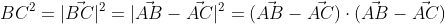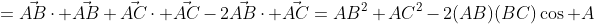Example 2. Angle bisector theorem.
In triangle ABC, let AD be the angle bisector of angle BAC meet BC at D, than AB:AC = BD:DC.
We can in fact prove this by sine law but this can be solved by vector.

Lemma. Consider parallelogram ABCD where AB = AC = CD. Then AD is perpendicular to angle  bisector of angle CAB.

Proof. Trivial by considering alternating angles.

Now consider triangle formed by two vectors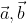. Let the angle bisector be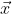. The angle bisector theorem is equivalent to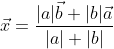.
Assume that this is true, we will show that x bisects the angle between two vectors.
Equating angle between a,x and b,x: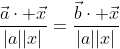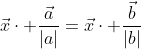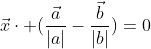Which is equivalent to the lemma above.

What is shown above is the existance of x only. We shall compute the dot product between x and the projection once in expanded form in order to show that it's really equal to zero.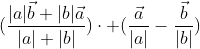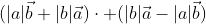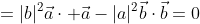Q.E.D.

Example 3. Medians of a triangle meet at one point.

Consider triangle formed by vector a,b. The three median vector is given by: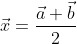,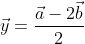and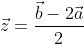. If they meet together, we can always find constantso that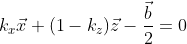,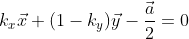, etc. because all of them form closed triangles if and only if the medians meet together. Now we solve that (quite complicated though) but all of us know that the constant is "one-third".

You may wonder why similar approach can't apply to proof on concurrence of angle bisector, it's because the constant for that case is varying, and solving the set of equation is harder because the representation of x,y,z are much more complicated.

In part II we will look for a more powerful tool, position vector, to solve more geometrical problems.